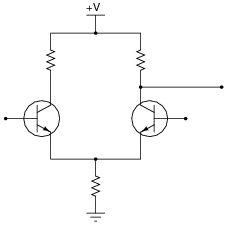Positive Feedback OpAmp Circuits

• Question 1

 Don’t just sit there! Build something!!

Learning to mathematically analyze circuits requires much study and practice. Typically, students practice by working through lots of sample problems and checking their answers against those provided by the textbook or the instructor. While this is good, there is a much better way.

You will learn much more by actually building and analyzing real circuits, letting your test equipment provide the “answers” instead of a book or another person. For successful circuit-building exercises, follow these steps:

1. Carefully measure and record all component values prior to circuit construction.
2. Draw the schematic diagram for the circuit to be analyzed.
3. Carefully build this circuit on a breadboard or other convenient medium.
4. Check the accuracy of the circuit’s construction, following each wire to each connection point, and verifying these elements one-by-one on the diagram.
5. Mathematically analyze the circuit, solving for all voltage and current values.
6. Carefully measure all voltages and currents, to verify the accuracy of your analysis.
7. If there are any substantial errors (greater than a few percent), carefully check your circuit’s construction against the diagram, then carefully re-calculate the values and re-measure.

Avoid using the model 741 op-amp, unless you want to challenge your circuit design skills. There are more versatile op-amp models commonly available for the beginner. I recommend the LM324 for DC and low-frequency AC circuits, and the TL082 for AC projects involving audio or higher frequencies.

As usual, avoid very high and very low resistor values, to avoid measurement errors caused by meter “loading”. I recommend resistor values between 1 kΩ and 100 kΩ.

One way you can save time and reduce the possibility of error is, to begin with a very simple circuit and incrementally add components to increase its complexity after each analysis, rather than building a whole new circuit for each practice problem. Another time-saving technique is to re-use the same components in a variety of different circuit configurations. This way, you won’t have to measure any component’s value more than once.

• Question 2

This is a very common opamp oscillator circuit, technically of the relaxation type:Explain how this circuit works, and what waveforms will be measured at points A and B. Be sure to make reference to RC time constants in your explanation.

• Question 3

Assume that the comparator in this circuit is only capable of “swinging” its output to within 1 volt of its power supply rail voltages. Calculate the upper and lower threshold voltages, given the resistor values shown:VUT = VLT =

• Question 4

One analogy used to explain and contrast negative feedback versus positive feedback is that of a round stone, placed on either a hilltop or a valley: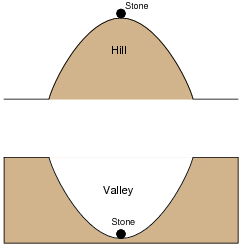The stability of the stone in each of these scenarios represents the stability of a specific type of electrical feedback system. Which of these scenarios represents negative feedback, which represents positive feedback, and why?

• Question 5

A student intends to connect a TL082 opamp as a voltage follower, to “follow” the voltage generated by a potentiometer, but makes a mistake in the breadboard wiring: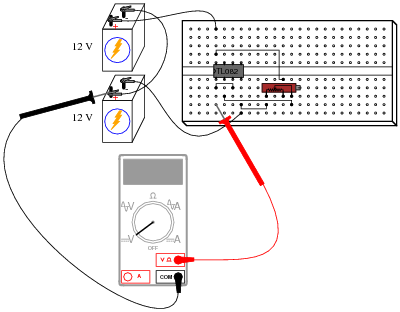Draw a schematic diagram of this faulty circuit, and determine what the voltmeter’s indication will be, explaining why it is such.

• Question 6

Determine the “trip” voltage of this comparator circuit: the value of input voltage at which the opamp’s output changes state from fully positive to fully negative or visa-versa:Now, what do you suppose would happen if the output were fed back to the noninverting input through a resistor? You answer merely has to be qualitative, not quantitative:For your information, this circuit configuration is often referred to as a Schmitt trigger.

• Question 7

A comparator is used as a high wind speed alarm in this circuit, triggering an audio tone to sound whenever the wind speed exceeds a pre-set alarm point:The circuit works well to warn of high wind speed, but when the wind speed is just near the threshold level, every little gust causes the alarm to briefly sound, then turn off again. What would be better is for the alarm to sound at a set wind speed, then stay on until the wind speed falls below a substantially lower threshold value (example: alarm at 60 km/h, reset at 50 km/h).

An experienced electronics technician decides to add this functionality to the circuit by adding two resistors: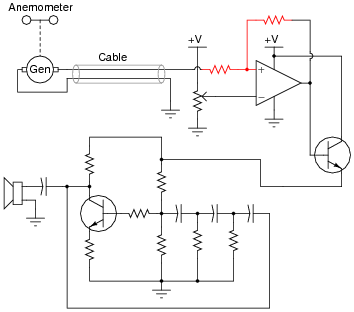Explain why this circuit alteration works to solve the problem.

• Question 8

Assume that the comparator in this circuit is capable of “swinging” its output fully from rail to rail. Calculate the upper and lower threshold voltages, given the resistor values shown: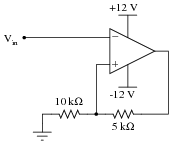VUT = VLT =

• Question 9

Assume that the comparator in this circuit is only capable of “swinging” its output to within 1 volt of its power supply rail voltages. Calculate the upper and lower threshold voltages, given the resistor values shown:VUT = VLT =

• Question 10

Assume that the comparator in this circuit is only capable of “swinging” its output to within 1 volt of its power supply rail voltages. Calculate the upper and lower threshold voltages, given the resistor values shown:VUT = VLT =

• Question 11

Comparators with positive feedback are sometimes referred to as Schmitt triggers. Suppose you needed a Schmitt trigger for a circuit you were building, but did not have any more integrated circuit comparators or op-amps to use. All you have available to you are discrete components. Is there any way you can think of to modify the following discrete transistor differential pair so that it behaves as a Schmitt trigger?